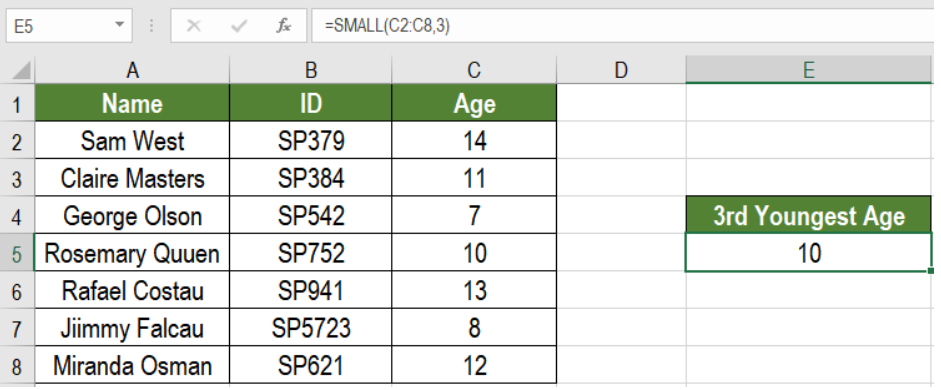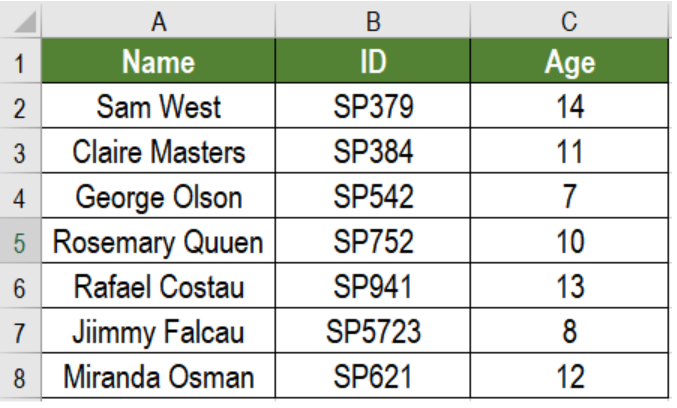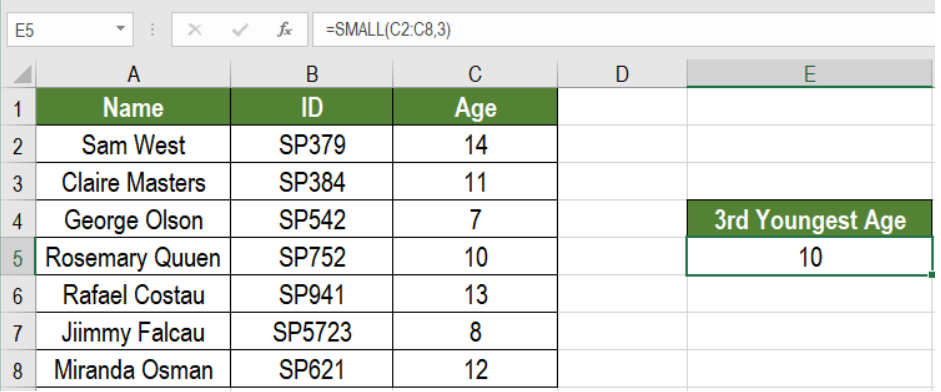Get instant live expert help with Excel or Google Sheets“My Excelchat expert helped me in less than 20 minutes, saving me what would have been 5 hours of work!”

#### Post your problem and you’ll get Expert help in seconds.

Your message must be at least 40 characters
Our professional Expert are available now. Your privacy is guaranteed.

# nth smallest value

Excel offers some cool ways to analyze data. One such tool is to find the nth smallest value from a data. It can be daunting to find the 2nd or 3rd smallest value manually if you have a large data set. However, Excel can help us to do that very easily. The SMALL function helps us to find the nth smallest value. In this tutorial, we will learn how to find the nth smallest value in Excel.Figure 1. How to find the Nth Smallest Value

## Formula

`=SMALL(range,nth)`

## Process

Here, we use the SMALL function. It works in an automatic way. From the syntax we see it uses two arguments range and nth. Range represents the range we want to find the nth smallest value for. We need to provide the range and an integer value n to the SMALL function. SMALL will return the desired nth value.

## Setting Up Data

The following example contains a children information database. Column A, B and C has the names, IDs and ages during the month of December.Figure 2. The Sample Data Set

To find the 3rd youngest age, we need to:

• Select cell E5 with our mouse.
• Write the formula `=SMALL(C2:D8,3) `to E5.
• Press Enter.Figure 3. Applying the Formula

This will show the 3rd youngest age, 10 in cell F5.

Most of the time, the problem you will need to solve will be more complex than a simple application of a formula or function. If you want to save hours of research and frustration, try our live Excelchat service! Our Excel Experts are available 24/7 to answer any Excel question you may have. We guarantee a connection within 30 seconds and a customized solution within 20 minutes.

### Did this post not answer your question? Get a solution from connecting with the expert.Another blog reader asked this question today on Excelchat:
Solution examplesI use a Google Sheet to keep track of prospective investors. The first tab functions as a master tab. Some of the columns I use to categorize the prospects. For example, if they are a candidate to lead for the next round, in the column, I would put a y for Yes. Then, I would like to have a separate tab that shows only those investors that I have flagged as possible lead investors.
Solved by I. H. in 40 minsI need to create a rows in Sheet 1 where Sheet 2 column c has values in Sheet 2 column D that are greater than .01 and leave out items in Sheet 1 where Sheet 3 column D are = 0 (without using a filter, then copy/paste).
Solved by I. Y. in 21 minscould someone breakdown this formula for me :D =ArrayFormula(IF(D\$5>=ROWS(B\$5:B5),INDEX(Sheet1!C\$6:C\$21,SMALL(IF(Sheet1!D\$6:D\$21=\$E\$5,ROW(Sheet1!C\$6:C\$21)-ROW(Sheet1!C\$6)+1),ROWS(B\$5:B5))),""))
Solved by C. C. in 40 minsI need a formula that looks for a specific name in three columns, takes all of the rows that match to get dates from another column. Then from the dates that match the rows with the names, take the second earliest date and generate it. The below formula is giving me the second date listed for the specific person instead of giving me the second earliest date. =IFERROR(INDEX('Input Data'!\$E\$5:\$E\$150,SMALL(IF(Tracker!\$B6='Input Data'!\$G\$5:\$I\$150,ROW('Input Data'!\$G\$5:\$I\$150)-ROW('Input Data'!\$G\$5)+1),2)), "N/A")
Solved by A. J. in 34 mins## Subscribe to Excelchat.coAnother blog reader asked this question today on Excelchat: